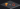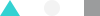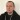# TensorFlow vs PyTorch

Author
Rafal Prońko
Published
Feb 11, 2019

*This is not my personal opinion but a framework overview. *

There are already countless blog posts on TensorFlow vs PyTorch out there, so why another comparison? We started using PyTorch at YND almost a year ago. Based on our experience, I’ll explain why we’re still using this framework instead of TensorFlow, despite changes in both of them. You’ll find a code comparison and conclusions on different aspects of development with each framework. Let’s get started!

## Framework introduction

TensorFlow was developed by Google Brain and is used by Google in both their research and production projects. Solutions based on this framework include:

• TensorFlow Lite
• TensorFlow.js
• Swift for TensorFlow
• TensorFlow probability
• Keras (high-level API)

The list of companies using TensorFlow comprises of globally recognized brands like Airbnb, Nvidia, Uber, SAP, Deepmind, Dropbox and eBay.

PyTorch was developed by Facebook. It was first used in their research team, and by now it has grown out to have a huge developer following.. There are also quite a few solutions built upon it:

• AllenNLP
• Fastai
• GPyTorch (Gaussian process library)
• Pyro (probabilistic programming language developed by Uber)

It was quickly adopted by companies and organisations such as Salesforce, Stanford and Udacity.

The best way to compare two frameworks is to write some code and take a closer look at it. Let’s try to build a simple classification with a built-in data set for fashion MNIST from Zalando. To make our life easier, for TensorFlow we will use a high-level API – Keras. For starters, let’s import the library:

 # for Pytorch import torch import torch.nn as nn import torchvision import torchvision.transforms as transforms # for tensorflow import tensorflow as tf from tensorflow import keras # other usefull library import numpy as np import matplotlib.pyplot as plt %matplotlib inline
view raw import_the_library hosted with ❤ by GitHub

Now it’s time to load the data.

Both PyTorch and TensorFlow offer built-in data load helpers. In both cases, there’s an easy and useful way to create the full pipeline for data (thanks to them, we can read, transform and create new data). We have DataSet class for PyTorch and tf.data for TensorFlow.

### PyTorch

In PyTorch, you can use a built-in module to load the data – dataset class. What’s more, you can easily use data augmentation –all you need to do is use appropriate dataset classes for image data transformation. The augmentation is created on the fly – you don’t have to do it in advance.

 # create the transformer for put the data to the tensor transforms = transforms.Compose([ transforms.ToTensor() ]) # load the train data - if not exists download it train_dataset_py = torchvision.datasets.FashionMNIST(root='/content/drive/My Drive/article/data/', train=True, transform=transforms, download=True) test_dataset_py = torchvision.datasets.FashionMNIST(root='/content/drive/My Drive/article/data/', train=False, transform=transforms, download=True)

### TensorFlow

In Keras, you can easily load the data, but if you want to create augmentation, you have to include an additional piece of code and save the images to the disk.

 # Load the data set fashion_mnist = keras.datasets.fashion_mnist #split to test and train (train_images_tf, train_labels_tf), (test_images_tf, test_labels_tf) = fashion_mnist.load_data()

The image range is different for each framework. In PyTorch, the image range is 0-1 while TensorFlow uses a range from 0 to 255. To use TensorFlow, we have to adapt the image range.

 train_images_tf = train_images_tf / 255.0 test_images_tf = test_images_tf / 255.0
view raw image_range hosted with ❤ by GitHub

Now we can see how the images look in PyTorch and TensorFlow.

 def imshowPytorch(img): npimg = img.numpy() plt.imshow(np.transpose(npimg, (1, 2, 0))) def imshowTensorFlow(img): plt.imshow(img)
view raw image_show hosted with ❤ by GitHub

For PyTorch, we have to change the tensor to the NumPy array – you can see it in imshowPyTorch. Here’s what it looks like…

view raw image_show_2 hosted with ❤ by GitHub

… and now for TensorFlow:

 imshowTensorFlow(train_images_tf) print(train_labels_tf)
view raw image_show_3 hosted with ❤ by GitHub

## Define a model

In both cases, defining a model is easy and looks pretty similar.

### PyTorch

To define a model in PyTorch, we have to create a special class where we can define each piece of the network/model. We can build it as a sequence of commands. The most important thing in this class is the __init__ method, in which we define the model and determine how it should transform the data.

For PyTorch, we also have two modes of the model: train and production. To put the model in the production mode, we just have to use method .eval() Once the model is in the production mode, some methods will be turned off automatically, such as dropout. To move it to the training mode, we have to use method .train() as train is the default mode.

 class NeuralNet(nn.Module): def __init__(self, num_of_class): super(NeuralNet, self).__init__() self.layer1 = nn.Sequential( nn.Conv2d(1, 16, kernel_size=5, stride=1, padding=2), nn.BatchNorm2d(16), nn.ReLU(), nn.MaxPool2d(kernel_size=2, stride=2)) self.layer2 = nn.Sequential( nn.Conv2d(16, 32, kernel_size=5, stride=1, padding=2), nn.BatchNorm2d(32), nn.ReLU(), nn.MaxPool2d(kernel_size=2, stride=2)) self.fc = nn.Linear(7 * 7 * 32, num_of_class) def forward(self, x): out = self.layer1(x) out = self.layer2(out) out = out.reshape(out.size(0), -1) out = self.fc(out) return out
view raw neural_net_pytorch hosted with ❤ by GitHub

### TensorFlow / Keras API

Keras doesn’t require creating a class, but the model is also created with the sequential method. Also, you don’t have to put the model in the production mode.

 modeltf = keras.Sequential([ keras.layers.Conv2D(input_shape=(28,28,1), filters=16, kernel_size=5, strides=1, padding="same", activation=tf.nn.relu), keras.layers.BatchNormalization(), keras.layers.MaxPooling2D(pool_size=2, strides=2), keras.layers.Conv2D(32, kernel_size=5, strides=1, padding="same", activation=tf.nn.relu), keras.layers.BatchNormalization(), keras.layers.MaxPooling2D(pool_size=2, strides=2), keras.layers.Flatten(), keras.layers.Dense(10, activation=tf.nn.softmax) ])
view raw neural_net_keras hosted with ❤ by GitHub

Next, we will create the model and define the loss function and optimizer.

## Creating a model in PyTorch & TensorFlow

### PyTorch

 modelpy = NeuralNet(10) criterion = nn.CrossEntropyLoss() optim = torch.optim.Adam(modelpy.parameters()) modelpy
view raw create_nn_pytorch hosted with ❤ by GitHub

### TensorFlow

view raw create_nn_tf hosted with ❤ by GitHub

As you can see, for TensorFlow/Keras API we have to compile the model, which means we need to create a computational graph. Meanwhile, the graph in PyTorch is created on the fly. Of course, it’s possible to create a model in TensorFlow without preparing the graph beforehand, but not as a built-in option – you have to use eager execution.

This difference affects the methods of model debugging. For PyTorch, you can use a Python debugging method like pdb or build it in PyCharm. For TensorFlow, there’s a special dedicated tool called TensorFlow debugger, which can be integrated with the TensorBordX.

## Running the network training

### PyTorch

For PyTorch, we have to define all steps – compute the output, the loss function and gradients and then update the network.

 %%time for e in range(10): # define the loss value after the epoch losss = 0.0 number_of_sub_epoch = 0 # loop for every training batch (one epoch) for images, labels in train_loader: #create the output from the network out = modelpy(images) # count the loss function loss = criterion(out, labels) # in pytorch you have assign the zero for gradien in any sub epoch optim.zero_grad() # count the backpropagation loss.backward() # learning optim.step() # add new value to the main loss losss += loss.item() number_of_sub_epoch += 1 print("step {}: loss: {}".format(e, losss / number_of_sub_epoch))
view raw training_pytorch hosted with ❤ by GitHub

### TensorFlow

Because Fashion MNIST in TensorFlow is an array with only two dimensions, we have to add the number of channels (in our case it’s just one):

 train_images_tf = train_images_tf.reshape(train_images_tf.shape, train_images_tf.shape, train_images_tf.shape, 1) %%time modeltf.fit(train_images_tf, train_labels_tf, epochs=10, batch_size=32)
view raw training_keras hosted with ❤ by GitHub

As you can see, the time of the training in both cases is similar to the function loss, which was predictable.

For training in Keras, we had to create only 2 lines of code instead of 12 lines in PyTorch. But we have to remember that Keras is a high-level API and not pure TensorFlow.

## Testing the models

### PyTorch

In PyTorch, it’s super simple. Use the name of the model model_name(X):

 correct = 0 total = 0 modelpy.eval() for images, labels in test_loader: outputs = modelpy(images) _, predicted = torch.max(outputs.data, 1) total += labels.size(0) correct += (predicted == labels).sum() print('Test Accuracy of the model on the {} test images: {}%'.format(total, 100 * correct / total))
view raw prediction_pytorch hosted with ❤ by GitHub

### TensorFlow

TensorFlow/Keras API is more similar to a well-known Machine Learning library, sklearn:

 test_images_tf = test_images_tf.reshape(test_images_tf.shape, test_images_tf.shape, test_images_tf.shape, 1) predictions = modeltf.predict(test_images_tf) correct = 0 for i, pred in enumerate(predictions): if np.argmax(pred) == test_labels_tf[i]: correct += 1 print('Test Accuracy of the model on the {} test images: {}%'.format(test_images_tf.shape, 100 * correct/test_images_tf.shape))
view raw prediction_keras hosted with ❤ by GitHub

In TensorFlow, we can evaluate the model one more way:

 test_loss, test_acc = modeltf.evaluate(test_images_tf, test_labels_tf) print('Test accuracy:', test_acc)
view raw prediction_keras_2 hosted with ❤ by GitHub

Because the models have similar loss value after the training, they also have a similar accuracy score.

### Serialization and Deployment

The ability to save and restore a model is extremely important. In both cases, you can save the model and restore it in just one command. After saving the model, we want to put it on production to be used by our services. And here comes the biggest difference.

In TensorFlow, we have a special method to that: TensorFlow Serving. This is a TensorFlow-integrated tool for serving and deployment, creating machine learning models and experiments. It also features production testing and supports model versioning. Tensorflow Serving uses gRPC.

PyTorch didn’t have any solution to cover deployment and serving processes. It wasn’t recommended to use in a production environment until the 1.0 version was announced. With an updated version, PyTorch combines research approach with production-ready features from Caffe2 and ONNX (Open Natural Network Exchange format, http://onnx.ai/), which ensures a stable model serving for a heavy load.

Now, let’s see how we can save the models.

 torch.save(modelpy, "/content/drive/My Drive/article/model.pt")
view raw save_torch hosted with ❤ by GitHub

Next we can easily load the model:

view raw load_torch hosted with ❤ by GitHub

Let’s check if the model was saved with the weight (didn’t lose the ability to predict):

 correct = 0 total = 0 for images, labels in test_loader: outputs = model_load_py(images) _, predicted = torch.max(outputs.data, 1) total += labels.size(0) correct += (predicted == labels).sum() print('Test Accuracy of the model on the {} test images: {}%'.format(total, 100 * correct / total))

### TensorFlow

view raw save_keras hosted with ❤ by GitHub

Both frameworks allow you to create a model in Python and export it to C++.

## API

As PyTorch is more tightly coupled with the native language than TensorFlow, it allows to develop things in a more dynamic and “Pythonic” way. A library-like design ensures seamless usage. TensorFlow on the other hand gives the impression of a much heavier tool with a separated computation part hidden behind a few interfaces (ex tf.Session). This makes PyTorch easier to learn and a popular first choice for beginners.

## Visualization

Computations performed with TensorFlow can be visualized by TensorBoard, a tool which helps to understand and optimize designed models. Among other features, it allows to show metrics, look up activated layers or plot learning progress.

PyTorch doesn’t provide any out-of-the-box solution. Charts and metrics are usually manually drawn by external plotting libraries or third party tools such as:

## Mobile

TensorFlow framework has a dedicated framework for mobile models – TensorFlow Lite. They have also built an easy-to-use converter between the full TensorFlow model and TensorFlow Lite.

PyTorch also allows you to convert a model to a mobile version, but you will need Caffe2 – they provide quite useful documentation for this.

## Quantisation of the model

Post-training quantization model is a well-known technique to reduce the model size. In TensorFlow, you can do it by converting the model to TensorFlow Lite as a parameter. Post-training quantisation quantized weights from floating-point to 8 bits of precision.In PyTorch, you have to use Glow. It’s able to convert floating-point-based networks into signed 8-bit integer networks.

## High-level API

In TensorFlow, you have two types of built-in API: high and low level. Our code example was built using the high-level API called Keras.

PyTorch has only low-level built-in API but you can try install and used sklearn like API - Skorch.

## AutoML

The new hot topic in deep learning is AutoML, a method to create deep neural networks automatically.Unfortunately for PyTorch, we have only an alpha-phase library for AutoML.

But for TensorFlow and Keras, we have the AutoKeras library. We can try to use this library for our data set.

## In summary

What’s the best solution? Which framework will work best for you? What I wanted to point out is that PyTorch and TensorFlow are fairly similar. However, there are a few reasons why we stick with the first one.

At YND, we test many solutions and PoC in a short period of time as we need to make simple changes quickly. We also have plenty of experience in development, so creating a simple API service isn't a problem. We also want to have the possibility of easy debugging without learning new semantics, and so on. This is why we are using PyTorch. In my personal opinion, this is a very fast and flexible framework to use in research.

### Tensorflow

Pros:

• Simple built-in high level API
• Tensorboard (easy to use visualisation tool)
• Simple serving method on production
• Very good documentation
• Easy mobile support

Cons:

• Static graph
• Debugging method
• Hard to make quick changes

### Pytorch

Pros:

• Python-like coding
• Dynamic graph
• Easy & quick editing
• Very good documentation available
• Plenty of projects out there which use Pytorch

Cons:

• Third party needed for visualisation
• API knowledge needed in Python to move to productionThis article was written by Rafal Prońko, a Data Scientist at YND.